# How to Set a Column Value to Null in SQL?

• Last Updated : 21 Aug, 2021

In this article, we will look into how you can set the column value to Null in SQL.

Firstly, let’s create a table using CREATE TABLE command:

Attention reader! Don’t stop learning now. Learn SQL for interviews using SQL Course  by GeeksforGeeks.

```-- create a table
CREATE TABLE students (Sr_No integer,Name varchar(20), Gender varchar(2));
-- insert some values
INSERT INTO students VALUES (1, 'Nikita', 'F');
INSERT INTO students VALUES (2, 'Akshit', 'M');
INSERT INTO students VALUES (3, 'Ritesh', 'F');
INSERT INTO students VALUES (4, 'Himani', 'F');
-- fetch some values
SELECT * FROM students ;```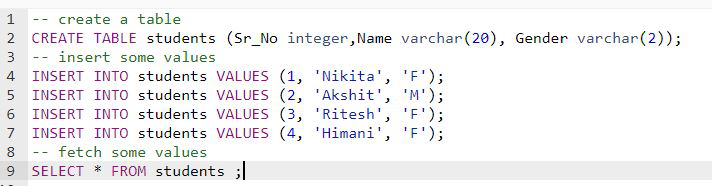The table would look like this:To UPDATE Column value, use the below command:

` UPDATE TABLE [TABLE_NAME]`

To set column value to NULL use syntax:

` update [TABLE_NAME] set [COLUMN_NAME] = NULL where [CRITERIA] `

Example: For the above table

```update students set Gender = NULL where Gender='F';
SELECT * FROM students ;```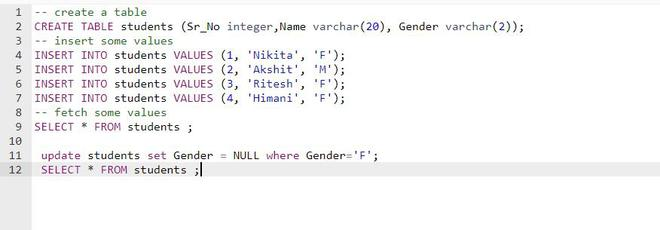Output:Column value can also be set to NULL without specifying the ‘where’ condition.

Example:

```update students set Gender = NULL;
SELECT * FROM students ;```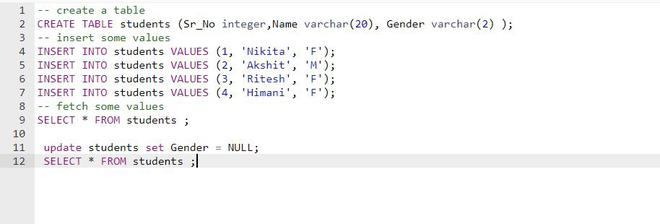Output:If you have set a constraint that a particular column value can not be NULL, and later try to set it as NULL, then it will generate an error.

Example:

```-- create a table
CREATE TABLE students (Sr_No integer,Name varchar(20), Gender varchar(2) NOT NULL);
-- insert some values
INSERT INTO students VALUES (1, 'Nikita', 'F');
INSERT INTO students VALUES (2, 'Akshit', 'M');
INSERT INTO students VALUES (3, 'Ritesh', 'F');
INSERT INTO students VALUES (4, 'Himani', 'F');
-- fetch some values
SELECT * FROM students ;```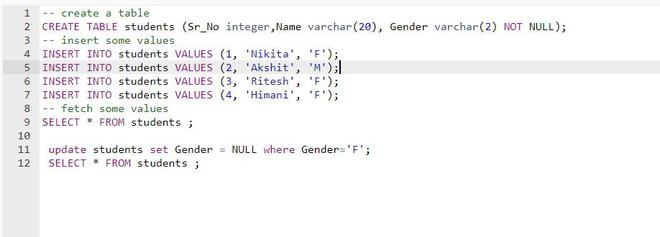Output: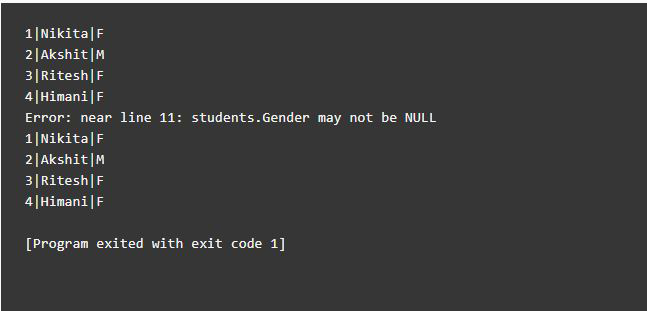ERROR: Gender may not be NULL.

My Personal Notes arrow_drop_up